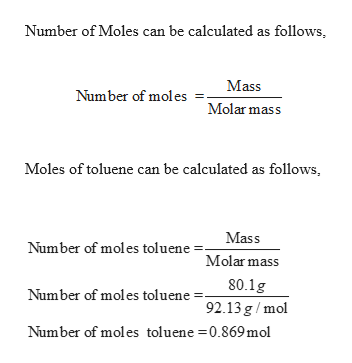# A solution is made using 80.1 g of toluene (MM = 92.13 g/mol) and 80.0 g of benzene (MM = 78.11 g/mol). What is the molality of the toluene in the solution?

Question
81 views

A solution is made using 80.1 g of toluene (MM = 92.13 g/mol) and 80.0 g of benzene (MM = 78.11 g/mol). What is the molality of the toluene in the solution?

check_circle

Step 1

Given,

Solution made using 80.1 g of toluene...help_outlineImage TranscriptioncloseNumber of Moles can be calculated as follows Mass Number of moles Molar mass Moles of toluene can be calculated as follows Mass Number of moles tolueneMolarmass 80.1g Number of moles toluene 92.13 g/mol Number of moles toluene =0.869 mol fullscreen

### Want to see the full answer?

See Solution

#### Want to see this answer and more?

Solutions are written by subject experts who are available 24/7. Questions are typically answered within 1 hour.*

See Solution
*Response times may vary by subject and question.
Tagged in

### Chemistry